# Solving Linear Equations Chapter 1 Solving Simple Equations

• Slides: 20Solving Linear Equations Chapter 1Solving Simple Equations and Multi. Step Equations I can solve multi-step linear equations using inverse operations.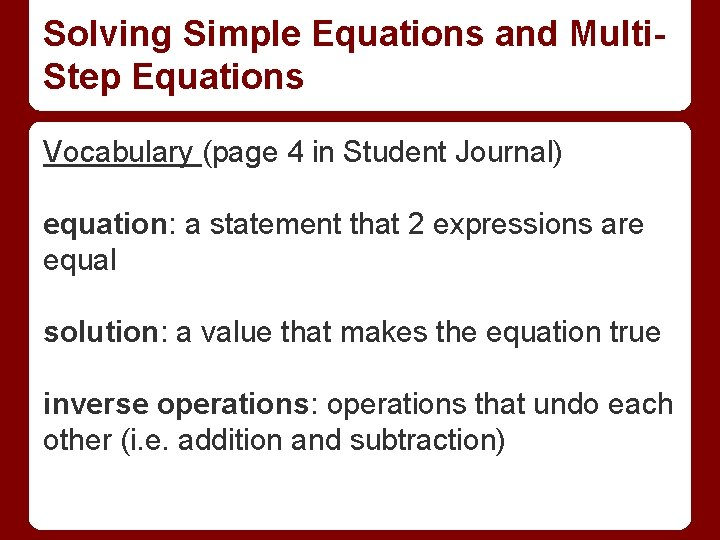Solving Simple Equations and Multi. Step Equations Vocabulary (page 4 in Student Journal) equation: a statement that 2 expressions are equal solution: a value that makes the equation true inverse operations: operations that undo each other (i. e. addition and subtraction)Solving Simple Equations and Multi. Step Equations equivalent equations: equations with the same solution(s)Solving Simple Equations and Multi. Step Equations Core Concepts (pages 4 and 5 in Student Journal) Addition Property of Equality if a = b, then a + c = b + c Subtraction Property of Equality if a = b, then a - c = b - c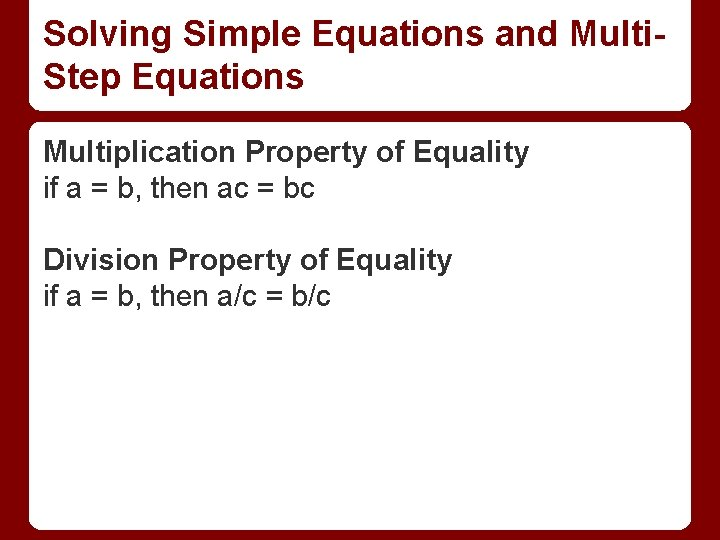Solving Simple Equations and Multi. Step Equations Multiplication Property of Equality if a = b, then ac = bc Division Property of Equality if a = b, then a/c = b/c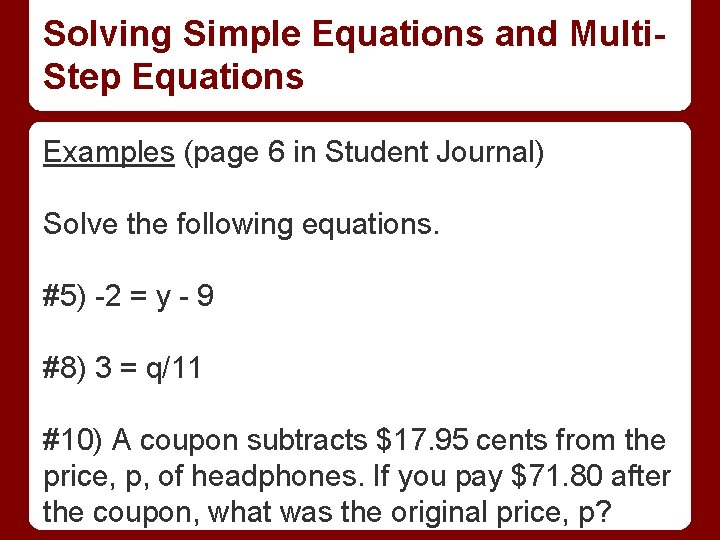Solving Simple Equations and Multi. Step Equations Examples (page 6 in Student Journal) Solve the following equations. #5) -2 = y - 9 #8) 3 = q/11 #10) A coupon subtracts \$17. 95 cents from the price, p, of headphones. If you pay \$71. 80 after the coupon, what was the original price, p?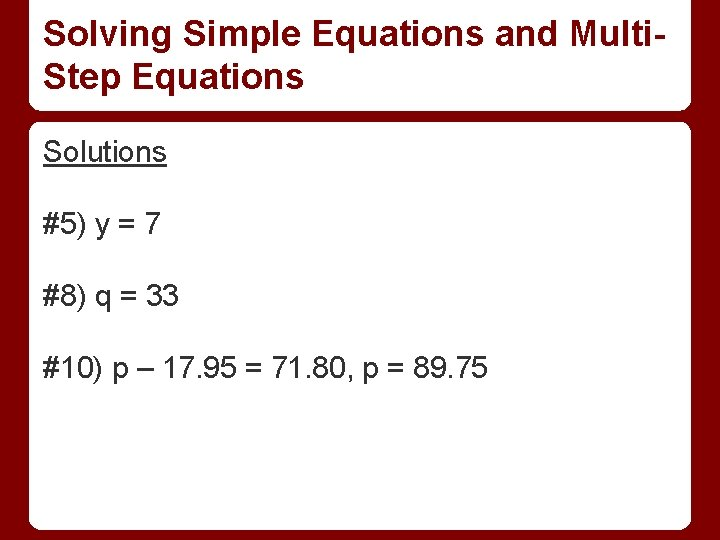Solving Simple Equations and Multi. Step Equations Solutions #5) y = 7 #8) q = 33 #10) p – 17. 95 = 71. 80, p = 89. 75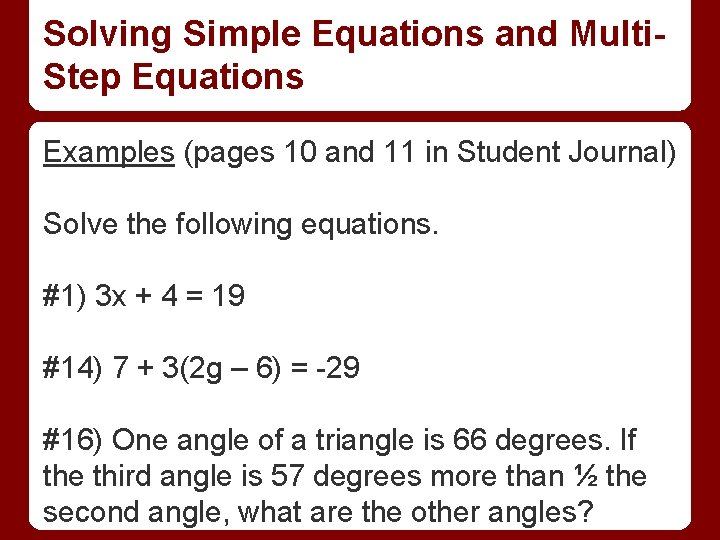Solving Simple Equations and Multi. Step Equations Examples (pages 10 and 11 in Student Journal) Solve the following equations. #1) 3 x + 4 = 19 #14) 7 + 3(2 g – 6) = -29 #16) One angle of a triangle is 66 degrees. If the third angle is 57 degrees more than ½ the second angle, what are the other angles?Solving Simple Equations and Multi. Step Equations Solutions #1) x = 5 #14) g = -3 #16) 66 + 1/2 x + 57 + x = 180, x = 38, 76 and 38 degrees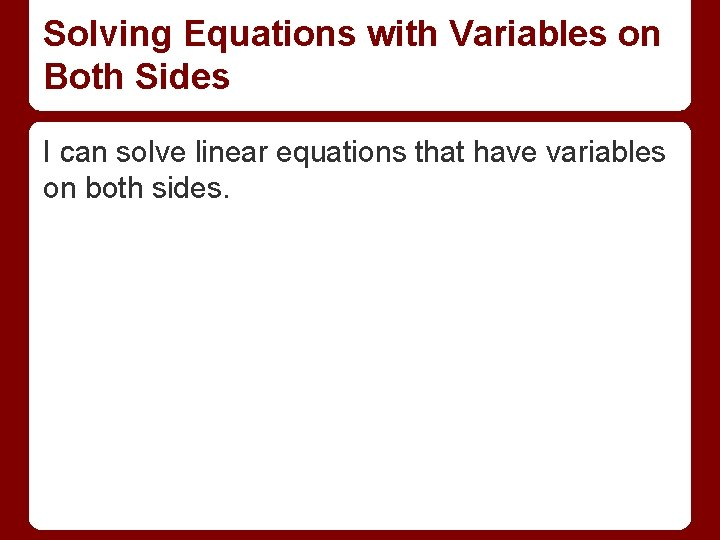Solving Equations with Variables on Both Sides I can solve linear equations that have variables on both sides.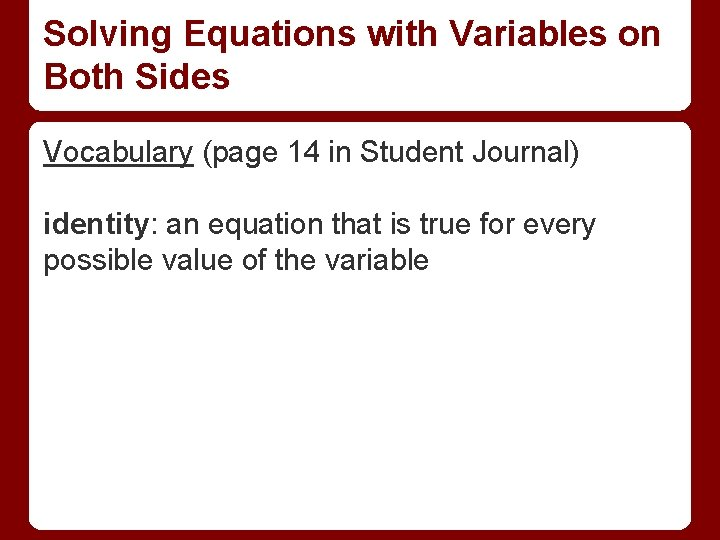Solving Equations with Variables on Both Sides Vocabulary (page 14 in Student Journal) identity: an equation that is true for every possible value of the variable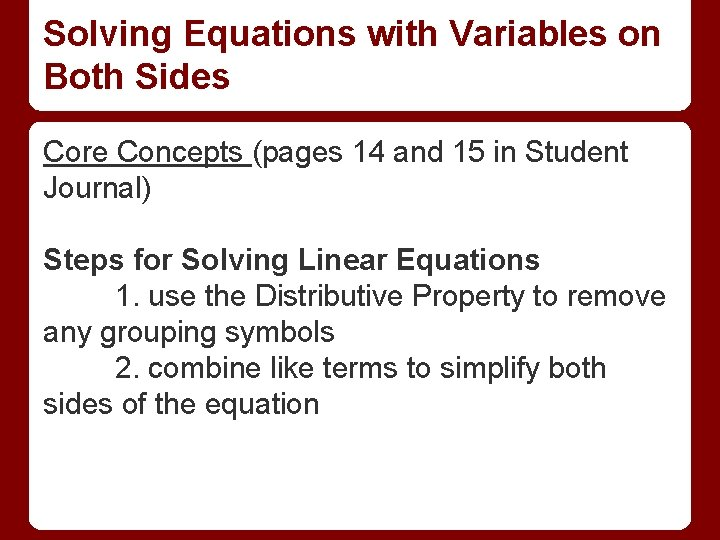Solving Equations with Variables on Both Sides Core Concepts (pages 14 and 15 in Student Journal) Steps for Solving Linear Equations 1. use the Distributive Property to remove any grouping symbols 2. combine like terms to simplify both sides of the equation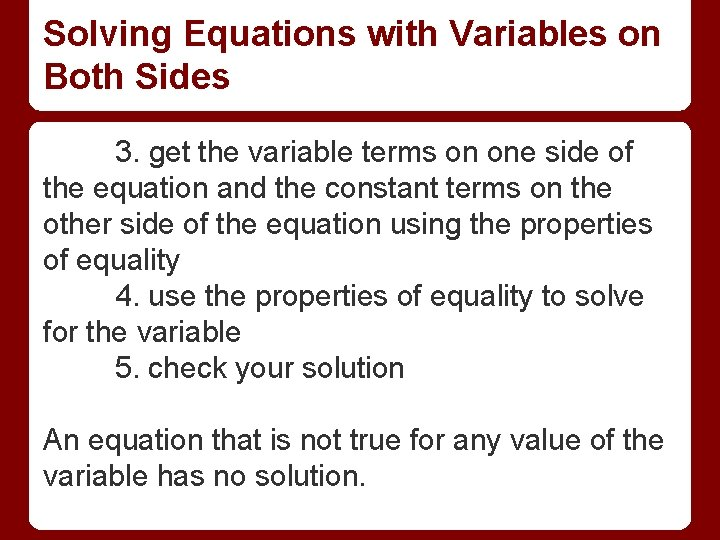Solving Equations with Variables on Both Sides 3. get the variable terms on one side of the equation and the constant terms on the other side of the equation using the properties of equality 4. use the properties of equality to solve for the variable 5. check your solution An equation that is not true for any value of the variable has no solution.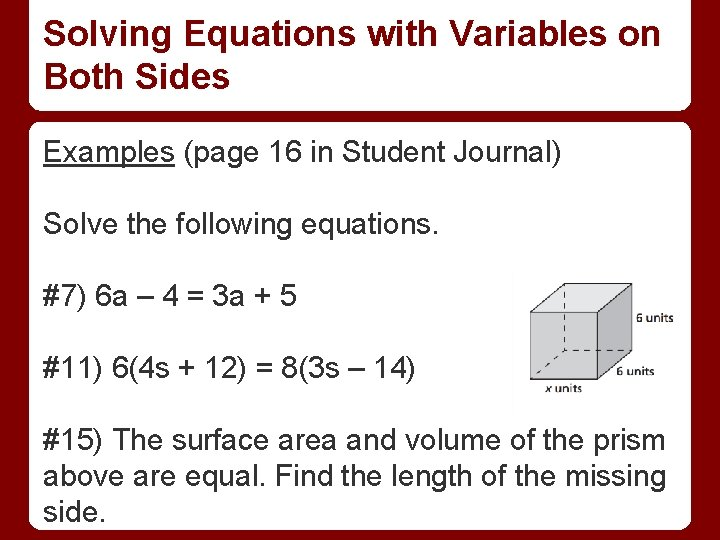Solving Equations with Variables on Both Sides Examples (page 16 in Student Journal) Solve the following equations. #7) 6 a – 4 = 3 a + 5 #11) 6(4 s + 12) = 8(3 s – 14) #15) The surface area and volume of the prism above are equal. Find the length of the missing side.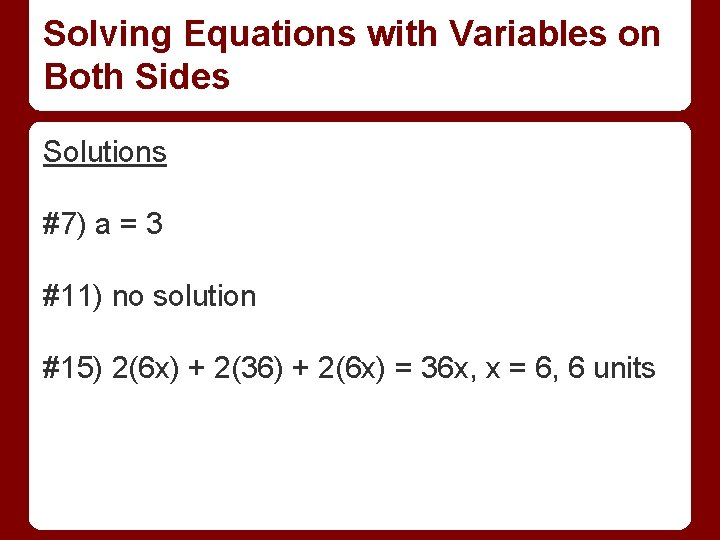Solving Equations with Variables on Both Sides Solutions #7) a = 3 #11) no solution #15) 2(6 x) + 2(36) + 2(6 x) = 36 x, x = 6, 6 unitsRewriting Equations and Formulas I can rewrite literal equations.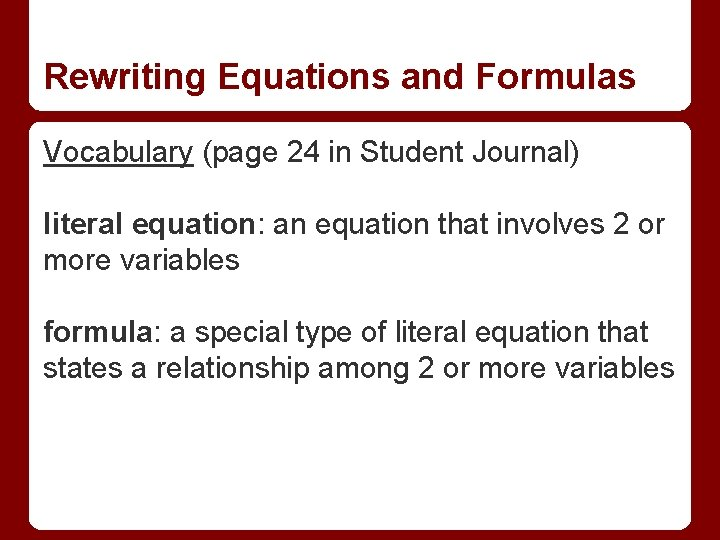Rewriting Equations and Formulas Vocabulary (page 24 in Student Journal) literal equation: an equation that involves 2 or more variables formula: a special type of literal equation that states a relationship among 2 or more variables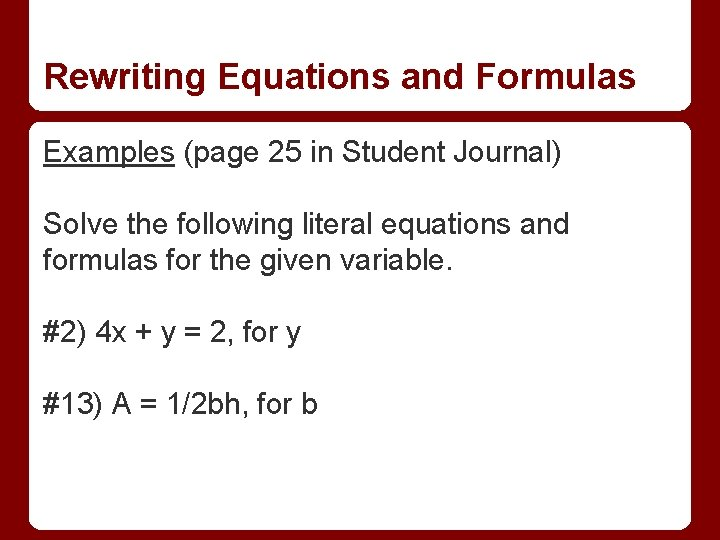Rewriting Equations and Formulas Examples (page 25 in Student Journal) Solve the following literal equations and formulas for the given variable. #2) 4 x + y = 2, for y #13) A = 1/2 bh, for bRewriting Equations and Formulas Solutions #2) y = -4 x + 2 #13) b = 2 A/h